# Common Core: 6th Grade Math : Identify Parts of an Expression Using Mathematical Terms: CCSS.Math.Content.6.EE.A.2b

## Example Questions

← Previous 1

### Example Question #1 : Identify Parts Of An Expression Using Mathematical Terms: Ccss.Math.Content.6.Ee.A.2b

Identify the sum in the equation below.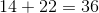Explanation:

The sum of an equation is the answer to an addition problem. In this case, the sum is.

### Example Question #2 : Identify Parts Of An Expression Using Mathematical Terms: Ccss.Math.Content.6.Ee.A.2b

Identify the sum in the equation below.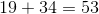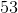Explanation:

The sum of an equation is the answer to an addition problem. In this case, the sum is### Example Question #3 : Identify Parts Of An Expression Using Mathematical Terms: Ccss.Math.Content.6.Ee.A.2b

Identify the term(s) in the equation below.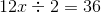All of the choices are correct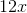All of the choices are correct

Explanation:

A term is a number or variable in an equation. Terms are separated by mathematical signs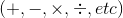### Example Question #4 : Identify Parts Of An Expression Using Mathematical Terms: Ccss.Math.Content.6.Ee.A.2b

Identify the product in the equation below.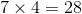Explanation:

The product of an equation is the answer to a multiplication problem. In this case, the product is### Example Question #5 : Identify Parts Of An Expression Using Mathematical Terms: Ccss.Math.Content.6.Ee.A.2b

Identify the product in the equation below.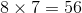Explanation:

The product of an equation is the answer to a multiplication problem. In this case, the product is### Example Question #6 : Identify Parts Of An Expression Using Mathematical Terms: Ccss.Math.Content.6.Ee.A.2b

Identify the factor(s) in the equation below.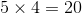BothandBothandBothandExplanation:

Factors are numbers that are multiplied together to get another number. In this case,andare factors of### Example Question #7 : Identify Parts Of An Expression Using Mathematical Terms: Ccss.Math.Content.6.Ee.A.2b

Identify the factor(s) in the equation below.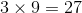BothandBothandBothandExplanation:

Factors are numbers that are multiplied together to get another number. In this case,andare factors of### Example Question #8 : Identify Parts Of An Expression Using Mathematical Terms: Ccss.Math.Content.6.Ee.A.2b

Identify the quotient(s) in the equation below.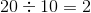BothandBothandExplanation:

The quotient of an equation is the answer to a division problem. In this case, the quotient is### Example Question #9 : Identify Parts Of An Expression Using Mathematical Terms: Ccss.Math.Content.6.Ee.A.2b

Identify the quotient(s) in the equation below.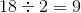BothandBothandExplanation:

The quotient of an equation is the answer to a division problem. In this case, the quotient is### Example Question #10 : Identify Parts Of An Expression Using Mathematical Terms: Ccss.Math.Content.6.Ee.A.2b

Identify the coefficient(s) in the equation below.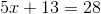BothandA coefficient is a number or symbol that is being multiplied by an unknown. In this case,is our unknown and it's being multiplied by, thusis our coefficient.